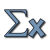Games
Problems
Go Pro!

# Sequences and Series

Reference > Mathematics > AlgebraExplanation of what a mathematical sequence is, with some examples, and questions.Instructions for finding the nth term of an arithmetic sequence, along with some examples of sequencesSolving problems involving arithmetic sequences.Explanation, formulas and problems about arithmetic series, along with the famous Carl Frederick Gauss story.Information about geometric sequences and how to find terms and ratios of geometric sequences.Information and problems about geometric series - series with a common ratioHow to find the sum of an infinite geometric series, when the ratio is between negative one and one.Sequences in which each term is defined in terms of the previous term (or terms)Like us on Facebook to get updates about new resources